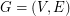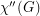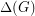Conjecture   A total coloring of a graphis an assignment of colors to the vertices and the edges ofsuch that every pair of adjacent vertices, every pair of adjacent edges and every vertex and incident edge pair, receive different colors. The total chromatic number of a graph,, equals the minimum number of colors needed in a total coloring of. It is an old conjecture of Behzad that for every graph, the total chromatic number equals the maximum degree of a vertex in,plus one or two. In other words,